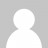# Selecting records from one table that dont have specific relationship in a second table

Question: How can you select records from one table that doesn’t have a specific ID in a second, many-to-many table?

In other words, imagine you have a questions table (A), categories table (B), and a many-to-many relationship link table (L).  This setup allows a question to have several categories.  How can you find questions that don’t have a specific category (say X)?

SELECT A.* FROM A JOIN L USING (question_id) WHERE L.category_id <> X;

This query will not meet the requirements because it will still return questions that have several categories in addition to the X category, since it will only filter the X value.

My way to handle this is to use the MySQL ‘NOT IN’ clause.

SELECT A.* FROM A WHERE A.question_id NOT IN (SELECT question_id FROM L WHERE category_id IN (X));

This query will retrieve all questions and related categories that don’t belong to category X.

Comments and/or different solutions are welcome.
Ilan.1.Burak says:

If you just need the questions, and not every single category:

SELECT A.*, SUM(IF(L.category_id = X,1,0)) as count_x LEFT JOIN L USING(question_id) WHERE count_x = 0 GROUP BY question_id;

2.rudy says:

SELECT A.* FROM A
LEFT OUTER JOIN L
ON L.question_id = A.question_id
AND L.category_id = X
WHERE L.category_id IS NULL

3.Hazan Ilan says:

This is a great query. Thanks

4.gloria says:

SELECT A.* FROM A
LEFT OUTER JOIN L
ON L.question_id = A.question_id
AND L.category_id = X
WHERE L.category_id IS NULL

I don’t understand that when we are taking the condition as L.category_id is NULL then Why are we taking the joining condition as L.Category_id=X

5.Hazan Ilan says:

The condition `(L.question_id = A.question_id AND L.category_id = X)`
at the ON clause force the left join to match only the records that DO have the X category. In other words, the records without the X category will be the records with null values. All you need, in order to get only those with the null values (records that not in category X), is to restrict the query to retrieve only the records with null by putting `L.category_id IS NULL` at the WHERE clause.

6.Amy says:

SELECT A.* FROM A
LEFT OUTER JOIN L
ON L.question_id = A.question_id
AND L.category_id = X
WHERE L.category_id IS NULL